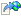﻿ComputeDerivedKey Method
 Pbkdf2 ComputeDerivedKey Method CryptSharp
Computes a derived key.

Namespace: CryptSharp.Utility
Assembly: CryptSharp (in CryptSharp.dll) Version: 2.0.0.0
Syntax

```public static byte[] ComputeDerivedKey(
KeyedHashAlgorithm hmacAlgorithm,
byte[] salt,
int iterations,
int derivedKeyLength
)```

#### Parameters

hmacAlgorithm
Type:System.Security.Cryptography KeyedHashAlgorithm
The HMAC algorithm to use, for exampleHMACSHA256. Make sure to setKey.
salt
Type:System Byte
The salt. A unique salt means a unique derived key, even if the original key is identical.
iterations
Type:System Int32
The number of iterations to apply.
derivedKeyLength
Type:System Int32
The desired length of the derived key.

The derived key.
See Also